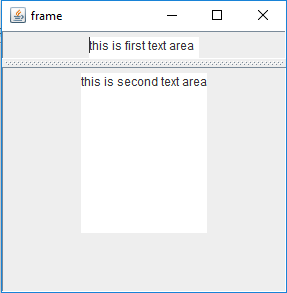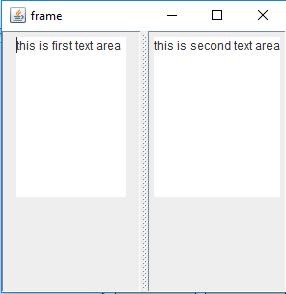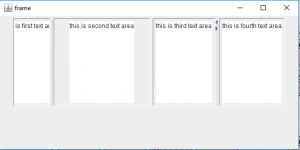# Java Swing | JSplitPane with Examples

JSplitPane is a part of Java Swing. JSplitPane is used to divide only two components. JSplitPane is to use resize the components . By using the JSplitPane the user can manually resize the component till its minimum size . JSplitPane can be of two types, one is the vertical and horizontal splitpane

Constructor of JSplitPane are:

1. JSplitPane() : creates a new split pane that is oriented horizontally
2. JSplitPane(int o) : creates a new split pane with orientation mentioned.
3. JSplitPane(int o, boolean r) : creates a new split pane with orientation and redrawing style mentioned.
4. JSplitPane(int o, boolean r, Component l, Component r) : creates a new split pane with orientation, redrawing style and left and right component mentioned.
5. JSplitPane(int o, Component l, Component r): creates a new split pane with orientation and left and right component mentioned.

Commonly used Functions of JSplitPane are :

1. getOrientation() : returns the orientation of the split pane
2. getRightComponent() : returns the right component of the splitpane
3. getTopComponent() : returns the top component of the splitpane
4. isContinuousLayout() :returns the continuous layout property
5. getBottomComponent() : returns the Bottom component of the splitpane
6. setRightComponent(Component c) : the right component of the splitpane is set to c
7. setLeftComponent(Component c) : the left component of the splitpane is set to c
8. setTopComponent(Component c) : the top component of the splitpane is set to c
9. setBottomComponent(Component c) : the bottom component of the splitpane is set to c
10. setUI(SplitPaneUI ui) : Sets the Look and feel object that renders this component.
11. setResizeWeight(double v) :Specifies how to distribute extra space when the size of the split pane changes.
12. setOneTouchExpandable(boolean n) : Sets the value of the oneTouchExpandable property, whichwhen true provides a UI widget that can collapse or expand the components on click
13. setDividerLocation(int l) : Sets the location of the divider.
14. setDividerSize(int n) : Sets the size of the divider.
15. setLastDividerLocation(int n) : Sets the last location the divider.
16. setDividerLocation(double p) : Sets the divider location as a percentage of the JSplitPane’s size.
17. setContinuousLayout(boolean n) : Sets the value of the continuousLayout property, which must be true for the child components to be continuously redisplayed .
18. remove(int index) : removes the Component at the specified index.
19. remove(Component c) : Removes the child component from the pane.
20. isOneTouchExpandable() : returns the oneTouchExpandable property.
21. isContinuousLayout() : returns the continuousLayout property.
22. getMinimumDividerLocation() : returns the minimum location of the divider .
23. getMaximumDividerLocation() : Returns the maximum location of the divider .
24. getDividerSize() : Returns the size of the divider.
25. getDividerLocation() : returns the last value passed to setDividerLocation.
26. addImpl(Component c, Object co, int i) : adds the specified component to this split pane.
27. setUI(SplitPaneUI ui) : sets the look and feel object that renders this component.
28. getUI() : returns the look and feel object that renders this component.
29. paramString() : returns a string representation of this JSplitPane.
30. getUIClassID() : returns the name of the Look and feel class that renders this component.
31. getAccessibleContext() : gets the AccessibleContext associated with this JSplitPane.

The Following programs will illustrate the use of JSplitPane
1. Program to create a horizontal JSplitPane to separate two text areas

 `// Java Program to create a horizontal JSplitPane  ` `// to separate two text areas ` `import` `javax.swing.event.*; ` `import` `java.awt.*; ` `import` `javax.swing.*; ` `class` `solve ``extends` `JFrame { ` ` `  `    ``// frame ` `    ``static` `JFrame f; ` ` `  `    ``// text areas ` `    ``static` `JTextArea t1, t2; ` ` `  `    ``// main class ` `    ``public` `static` `void` `main(String[] args) ` `    ``{ ` `        ``// create a new frame ` `        ``f = ``new` `JFrame(``"frame"``); ` ` `  `        ``// create a object ` `        ``solve s = ``new` `solve(); ` ` `  `        ``// create a panel ` `        ``JPanel p1 = ``new` `JPanel(); ` `        ``JPanel p = ``new` `JPanel(); ` ` `  `        ``// create text areas ` `        ``t1 = ``new` `JTextArea(``10``, ``10``); ` `        ``t2 = ``new` `JTextArea(``10``, ``10``); ` ` `  `        ``// set texts ` `        ``t1.setText(``"this is first text area"``); ` `        ``t2.setText(``"this is second text area"``); ` ` `  `        ``// add text area to panel ` `        ``p1.add(t1); ` `        ``p.add(t2); ` ` `  `        ``// create a splitpane ` `        ``JSplitPane sl = ``new` `JSplitPane(SwingConstants.HORIZONTAL, p1, p); ` ` `  `        ``// add panel ` `        ``f.add(sl); ` ` `  `        ``// set the size of frame ` `        ``f.setSize(``300``, ``300``); ` ` `  `        ``f.show(); ` `    ``} ` `} `

Output :2.Program to create a Vertical JSplitPane to separate two text areas

 `// Java Program  to create a vertical  ` `// JSplitPane to separate two text areas ` `import` `javax.swing.event.*; ` `import` `java.awt.*; ` `import` `javax.swing.*; ` `class` `solve ``extends` `JFrame { ` ` `  `    ``// frame ` `    ``static` `JFrame f; ` ` `  `    ``// text areas ` `    ``static` `JTextArea t1, t2; ` ` `  `    ``// main class ` `    ``public` `static` `void` `main(String[] args) ` `    ``{ ` `        ``// create a new frame ` `        ``f = ``new` `JFrame(``"frame"``); ` ` `  `        ``// create a object ` `        ``solve s = ``new` `solve(); ` ` `  `        ``// create a panel ` `        ``JPanel p1 = ``new` `JPanel(); ` `        ``JPanel p = ``new` `JPanel(); ` ` `  `        ``// create text areas ` `        ``t1 = ``new` `JTextArea(``10``, ``10``); ` `        ``t2 = ``new` `JTextArea(``10``, ``10``); ` ` `  `        ``// set texts ` `        ``t1.setText(``"this is first text area"``); ` `        ``t2.setText(``"this is second text area"``); ` ` `  `        ``// add text area to panel ` `        ``p1.add(t1); ` `        ``p.add(t2); ` ` `  `        ``// create a splitpane ` `        ``JSplitPane sl = ``new` `JSplitPane(SwingConstants.VERTICAL, p1, p); ` ` `  `        ``// set Orientation for slider ` `        ``sl.setOrientation(SwingConstants.VERTICAL); ` ` `  `        ``// add panel ` `        ``f.add(sl); ` ` `  `        ``// set the size of frame ` `        ``f.setSize(``300``, ``300``); ` ` `  `        ``f.show(); ` `    ``} ` `} `

Output :2. Java Program to create nested JSplitPane, one of them is one Touch Expandable

 `// Java Program to create nested JSplitPane,  ` `// one of them is one Touch Expandable ` `import` `javax.swing.event.*; ` `import` `java.awt.*; ` `import` `javax.swing.*; ` `class` `solve ``extends` `JFrame { ` ` `  `    ``// frame ` `    ``static` `JFrame f; ` ` `  `    ``// text areas ` `    ``static` `JTextArea t1, t2, t3, t4; ` ` `  `    ``// main class ` `    ``public` `static` `void` `main(String[] args) ` `    ``{ ` `        ``// create a new frame ` `        ``f = ``new` `JFrame(``"frame"``); ` ` `  `        ``// create a object ` `        ``solve s = ``new` `solve(); ` ` `  `        ``// create a panel ` `        ``JPanel p1 = ``new` `JPanel(); ` `        ``JPanel p = ``new` `JPanel(); ` `        ``JPanel p2 = ``new` `JPanel(); ` `        ``JPanel p3 = ``new` `JPanel(); ` ` `  `        ``// create text areas ` `        ``t1 = ``new` `JTextArea(``10``, ``10``); ` `        ``t2 = ``new` `JTextArea(``10``, ``10``); ` `        ``t3 = ``new` `JTextArea(``10``, ``10``); ` `        ``t4 = ``new` `JTextArea(``10``, ``10``); ` ` `  `        ``// set texts ` `        ``t1.setText(``"this is first text area"``); ` `        ``t2.setText(``"this is second text area"``); ` `        ``t3.setText(``"this is third text area"``); ` `        ``t4.setText(``"this is fourth text area"``); ` ` `  `        ``// add text area to panel ` `        ``p1.add(t1); ` `        ``p.add(t2); ` `        ``p2.add(t3); ` `        ``p3.add(t4); ` ` `  `        ``// create a splitpane ` `        ``JSplitPane sl = ``new` `JSplitPane(SwingConstants.VERTICAL, p1, p); ` `        ``JSplitPane s2 = ``new` `JSplitPane(SwingConstants.VERTICAL, p2, p3); ` ` `  `        ``// set Orientation for slider ` `        ``sl.setOrientation(SwingConstants.VERTICAL); ` `        ``s2.setOrientation(SwingConstants.VERTICAL); ` ` `  `        ``s2.setOneTouchExpandable(``true``); ` ` `  `        ``// set divider location ` `        ``sl.setDividerLocation(``70``); ` ` `  `        ``// set Layout for frame ` `        ``f.setLayout(``new` `FlowLayout()); ` ` `  `        ``// add panel ` `        ``f.add(sl); ` `        ``f.add(s2); ` ` `  `        ``// set the size of frame ` `        ``f.setSize(``600``, ``300``); ` ` `  `        ``f.show(); ` `    ``} ` `} `

Output:Note : This above programs might not run in an online compiler please use an offline IDE

Attention reader! Don’t stop learning now. Get hold of all the important DSA concepts with the DSA Self Paced Course at a student-friendly price and become industry ready.

My Personal Notes arrow_drop_upCheck out this Author's contributed articles.

If you like GeeksforGeeks and would like to contribute, you can also write an article using contribute.geeksforgeeks.org or mail your article to contribute@geeksforgeeks.org. See your article appearing on the GeeksforGeeks main page and help other Geeks.

Please Improve this article if you find anything incorrect by clicking on the "Improve Article" button below.

Improved By : Akanksha_Rai

Article Tags :
Practice Tags :

Be the First to upvote.

Please write to us at contribute@geeksforgeeks.org to report any issue with the above content.